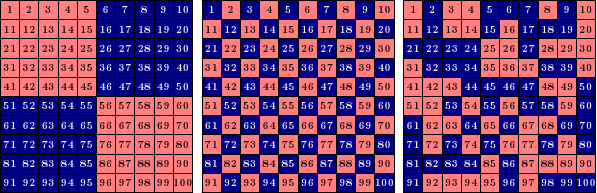# A Balancing Fact

Enough geometry already! Let’s switch gears and explore an interesting phenomenon in balanced grid coloring:

Problem: The numbers 1,2,…,100 are written in order in a $$10\times 10$$ grid. Some grid cells are colored red, and the rest blue, in such a way that exactly half the cells in each row and each column are red (call this a balanced coloring). Show that the sum of the numbers in red cells equals the sum of the numbers in blue cells.Examples of balanced grids: each grid has five red and five blue cells in each row and column. By the problem above, the red numbers and blue numbers add to the same total in each grid.

How can we understand this question? Notice that each row needs five red and five blue cells, but the values in each row do not need to balance. Indeed, the first row of the first example above is very skewed toward blue: $$\text{red}=1+2+3+4+5=15$$ and $$\text{blue}=6+7+8+9+10=40$$. In fact, every row in that example is very skewed to one side or the other! But the fact that every column also needs five red and five blue numbers somehow forces these skewed row sums to cancel each other out. This is essentially what the problem asks us to prove.

### A (Not So Great) Start

Here’s one idea: let’s just test all the balanced grid colorings! There can’t be too many, right? Let’s start small. How many balanced $$2\times 2$$ grid colorings are there? (Each row needs 1 red and 1 blue.) Just two: the first cell is either red or blue, and everything else is determined from there (cf. binary sudoku). And each of these has a red sum and a blue sum of 5, so we’ve verified the problem for $$2\times 2$$ grids. What about $$4\times 4$$? With a little work, you can compute by hand that there are 90 balanced colorings of a $$4\times 4$$ grid (try this!). That’s already a lot of cases to check. For $$10\times 10$$ grids, it turns out that there are 6736218287430460752 balanced colorings! Maybe it’s time to look for a different method.

### A Solution

Here’s a method that does work. Start with a zero in each grid cell; the red and blue sums are certainly equal (they’re both zero). Now we’ll modify the values one row or column at a time. If we add 1 to each cell in a column, then the red and blue sums each increase by 5 (because each column is balanced), so the sums remain equal. Do this once in the first column, twice in the second column, and so on, resulting in the middle grid below (which must therefore have equal red and blue sums):Adding the same number to each cell in a row or column of a balanced grid keeps the red and blue sums equal. Applying this process to an initially all-zero grid yields the desired grid of 1,2,...,100 and solves the problem.

Now modify the rows: add 10 to the second row, 20 to the third row, etc., finally ending up with the numbers 1,2,…,100 in order. Each step preserves the fact that the red and blue sums match, so we’re done! Notice that this argument works for any even grid size; 10 is not special here.

1.Michael P says: